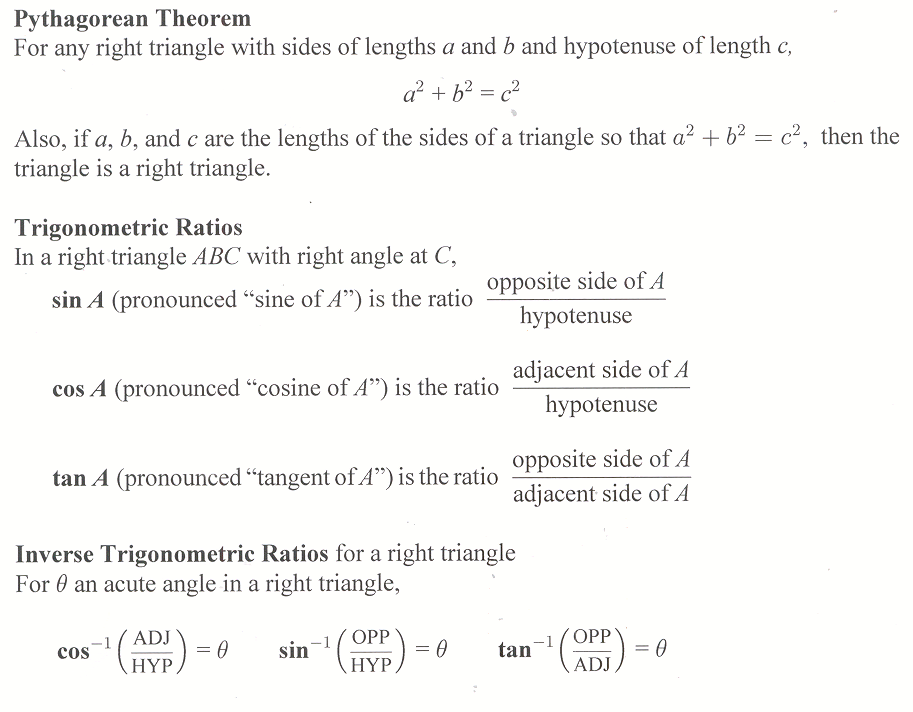# Section 7.5: Right-Triangle Trigonometry

## 7.5 Outline

1. Pythagorean theorem
2. Trigonometric ratios
1. sine
2. cosine
3. tangent
3. Inverse trigonometric ratios
1. calculator calculating
2. inverse trigonometric ratios
1. inverse tangent
2. inverse cosine
3. inverse sine
4. Applications
1. angle of elevation
2. angle of depression

## 7.5 Essential Ideas